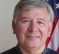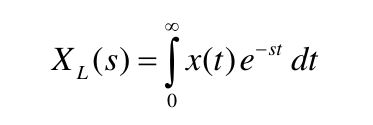## What is the difference between the Fourier transform of an analytic signal and the one-sided Fourier transform?Started by 7 years ago6 replieslatest reply 7 years ago7767 views

The spectrum of an analytic signal equals twice the spectrum of its real part for positive frequencies and it is zero for negative frequencies. Now, I wonder: When I take the one-sided Fourier Transform of the real part of the analytic signal, would this be equal to the Fourier transform of the analytic signal?

The one-sided Fourier transform has only positive frequency components and its amplitude is twice the amplitude of the double-sided Fourier transform. So, I don't see a difference between the one-sided Fourier transform of a real signal x(t) and the Fourier transform of its analytic signal z(t) = x(t) + H(x(t))

[ - ]By looking into the DFT equation:

$$X[m] = \sum_{n=0}^{N-1} x[n] e^{-j2\pi m \frac{n}{N}}$$

where N is the number of input samples, and m is the frequency bins.

Let's elaborate on how the m defines the frequency bins. If the sampling frequency (fs ) is 8 kHz, and m is 8, consequently, the frequency resolution of the DFT is $$\frac{f_{s}}{m}=8000/8=1000$$.

The frequency bins will be located at [0 1000 2000 3000 4000 5000 6000 7000] which are 8 frequency bins separated by 1 kHz step size. However, it is possible to alter the frequency bins in such a way to start from negative frequency instead of DC (0Hz). The new bins are [-4000 -3000 -2000 -1000 0 1000 2000 3000] which are 8 frequency bins separated by 1 kHz step size as well.

In summary, the equation itself will compute the complete spectrum at the frequency bins which you define.

[ - ]I don't understand this statement:

"The one-sided Fourier transform has only positive frequency components and its amplitude is twice the amplitude of the double-sided Fourier transform."

Perhaps it would be good to define "one-sided Fourier Transform".  I couldn't find a definition of such a thing.

Now, perhaps you are referring to the Fourier Transform of a function that is zero for t<0?  That's fine but the transform remains the Fourier Transform.  It's only the function being transformed that's "one-sided".  At that, you may find that the transform doesn't exist and will quickly get into discussion re: Lapalace Transform. .. but I digress perhaps.

"I don't see a difference between the one-sided Fourier transform of a real signal x(t)"

A real signal x(t) doesn't have the property that it has no negative-frequency components.  So, I'm still concerned about the use of the term "one-sided Fourier Transform".  I think that's the confusion here.

Maybe you mean this:

1) One can define an analytic signal derived from a real signal x(t) as: z(t) = x(t) + H(x(t))

2) One can compute the Fourier Transform of z(t) = Z(f) to find that it has no negative-frequency components.

3) One can compute the Fourier Transform of x(t) = X(f) to find that it has negative and positive-frequency components.

4) One can zero out the negative frequency components of X(f) by multiplying it by a step function in frequency.  This results in a "one-sided" function of frequency but that's a function and not a transform.  I suppose you could (at least conceptually) convolve the Inverse Fourier Transform of the frequency step with x(t).  The result isn't much like the original x(t).

The likelihood is that we confuse "the Fourier Transform of x(t)" with the more-correct: "the result X(f) of Fourier Transforming x(t)" which is clearly a function and not a transform (i.e. an operation).

There's a pretty good treatment right here:

https://www.dsprelated.com/freebooks/mdft/Analytic...

[ - ]Thanks Fred, this really helps!

But I am still a little bit confused:

Since you asked for a definition of the one-sided Fourier transform, I started looking for one. And I couldn't find one, which is weird, because I have been using it in class. But I have found a definition of a one-sided Laplace transform, which is a transform only for causal signals:Now, when replacing s with jw, this should give the one-sided Fourier transform.

On page 25 of this book, there is a figure that shows the frequency spectrum of a bandpass signal and its analytic signal. The first figure (a), depicts the two-sided Fourier transform of the bandpass signal, I think. Maybe I am using the term 'Fourier transform' wrong, and I must correct it to frequency spectrum. When I don't want the frequency spectrum to have negative frequency components, then I would cancel the negative components and multiply the positive frequency components by 2. This, I figured, would be achieved through the one-sided Fouier transform. But I can be completely wrong here.

[ - ]taking a simple example (cos) its analytic is(cos+jsin).

any scaling is matter of design but matlab keeps unity of amplitude:

x = cos(2*pi*(0:1023)*.01);

y = hilbert(x);

plot(x);hold

plot(real(y)); %cos same as x

plot(imag(y)); %sin

[ - ]"Du hast entweder eine Seite erreicht, die nicht angezeight werden kann, oder die Anqeigebechraenkung fuer dieses Buch erreicht" (umlauts mangled).

My German is only good enough to tell that it's an error about a book.

I don't want it to be "jump on Lucky_12 day", but the notion of a "causal signal" doesn't really make sense.  A signal just is, for whatever time it exists in.  There may be a causal or non-causal system in the mix, generating output signals that come after or before their cause, but that's a different matter.

I'm actually not sure why the Laplace transform is typically defined as being from $$t = 0$$ to $$\infty$$ vs. the Fourier transform range.  I kind of wish I knew, because there's some handy math that you can do if you redefine things.

[ - ]Well, when one asks a question about mathematics and definitions then I try to stick with the definitions as well as I might.  I'm not trying to be critical of YOU, just critical in my use of language.  I'm not a mathematician; just a user of math.  Anyway, one of the few references that I could Google was this one:

https://web.stanford.edu/class/ee102/lectures/four...

And, "The first figure (a), depicts the two-sided Fourier transform of the bandpass signal" I'm sorry but I can only sort of get the German as I have no capability there.  Anyway, I think calling it a "two-sided Fourier Transform" has a couple of problems:  T

- The first problem is calling it a Fourier Transform when, in fact, it might be more properly called "the result of Fourier Transforming xBP(t)".  So, I'm being picky and only willing to call something the "Fourier Transform" = a mapping transformation and not the mapped result.  This then leads to a bit more precise treatment.  It's a function and not an operation.  We will confuse functions and operations here very easily. The integral equation you used is an example of a "single-sided" integral I suppose whether x(t) is zero for t<0 or not.  Anyway, it's part of the definition of the operation or transformation.  But the example of figure (b) is a "single sided" function of frequency.

- The other problem is calling it "two-sided".  That rather implies that there may be a "one-sided" version.  figure (b) isn't a "one-sided" version of the Fourier Transform but it might be referred to as a "one-sided" version of a function. We just can't magically remove the negative frequency components without knowing how it was done. More properly, it would be the product of a step function and the original "two-sided" function.  That's a *new* function with mathematical meaning.  And, in general terms at least, there may be an operation in the opposite transform domain that's equivalent.  That suggests there is an operation in time (convolution) that's equivalent to multiplying by a step in frequency.

Now I will try to rephrase your initial concern:

"I don't see a difference between the one-sided Fourier transform of a real signal x(t) and the Fourier transform of its analytic signal z(t) = x(t) + H(x(t))"

I hope this works... here is my paraphrasing:

"Where x(t) <> X(f)) means "the Fourier Transform pair" where
X(f) is the Fourier Transformed result of x(t)
and
x(t) is the Inverse Fourier Transformed result of X(f)

I don't see a difference between the result of multiplying X(f) by S(f) where S(f)=0 for f<0 and S(f)=1.0 for f>=0

and the Fourier transform of z(t) = x(t) + H(x(t)) <> X(f) + G(f)
[end]

Perhaps one shouldn't see a difference.  But I believe there is one:

First of all, the negative frequency components of X(f) are cancelled by the negative frequency components of G(f), right?
And then the positive frequency components of G(f) add to the positive frequency components of X(f).
I think there will surely be a difference.

We have X(f_) + G(f_) = 0
We also have X(f+) + G(f+) = ~something that's not X(f+) alone...